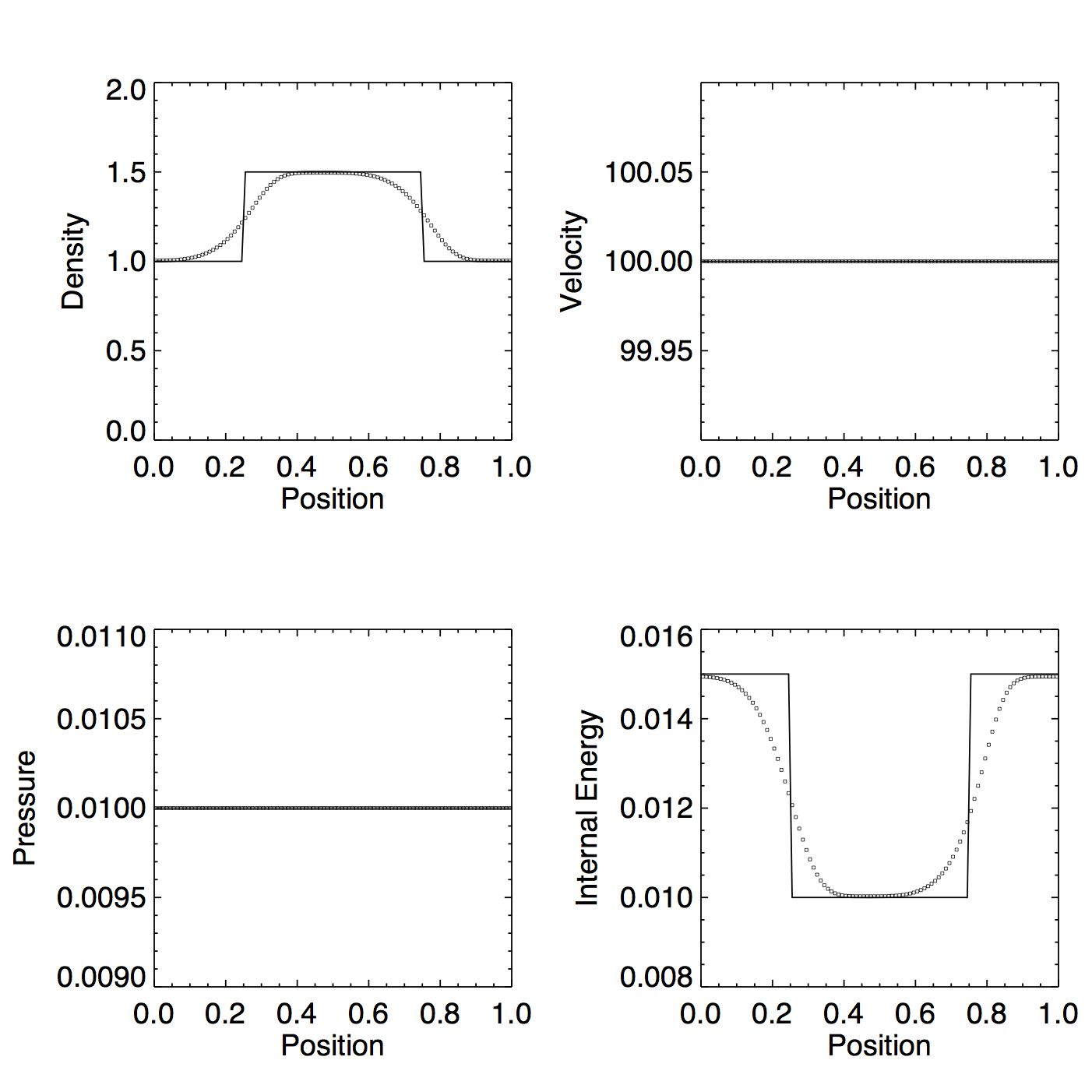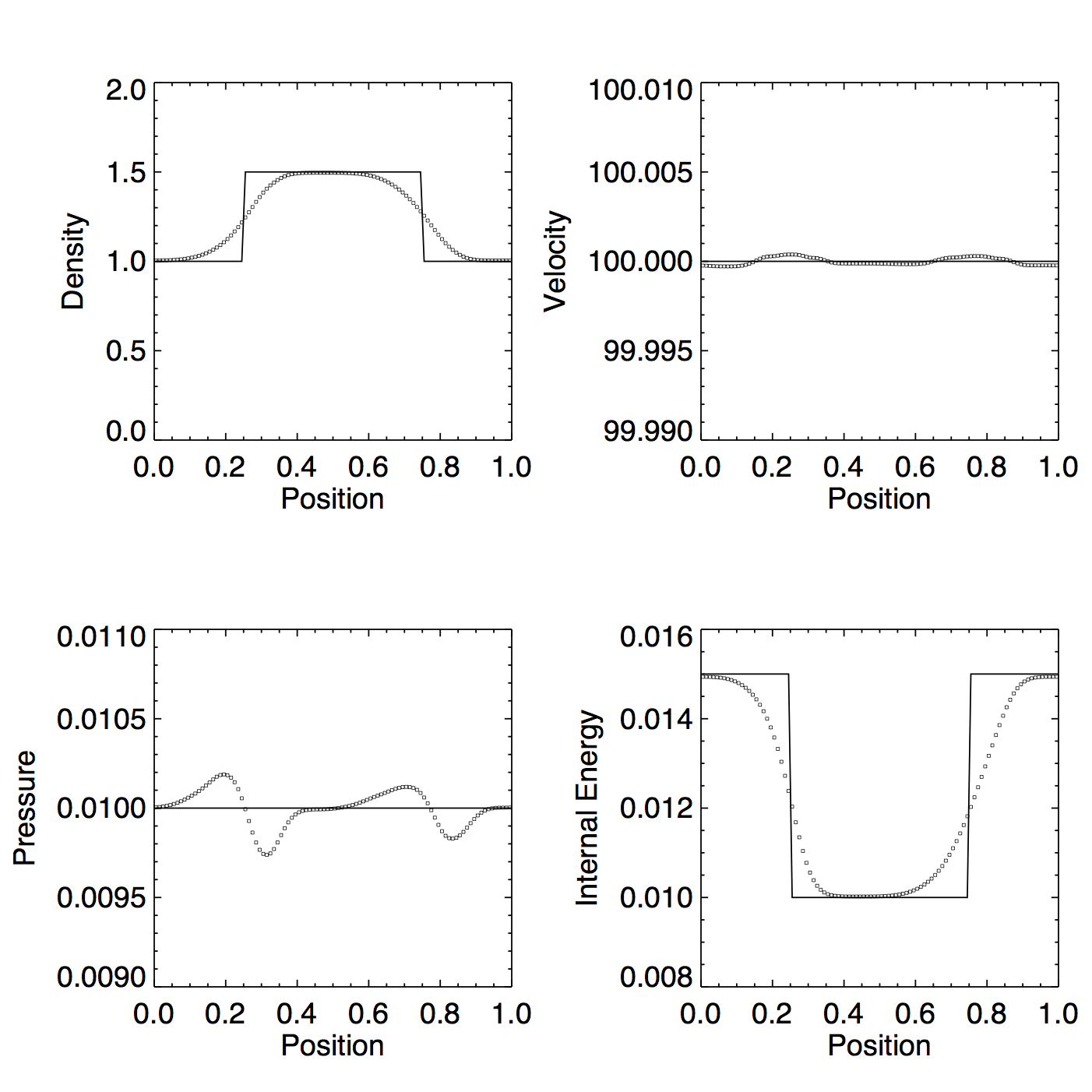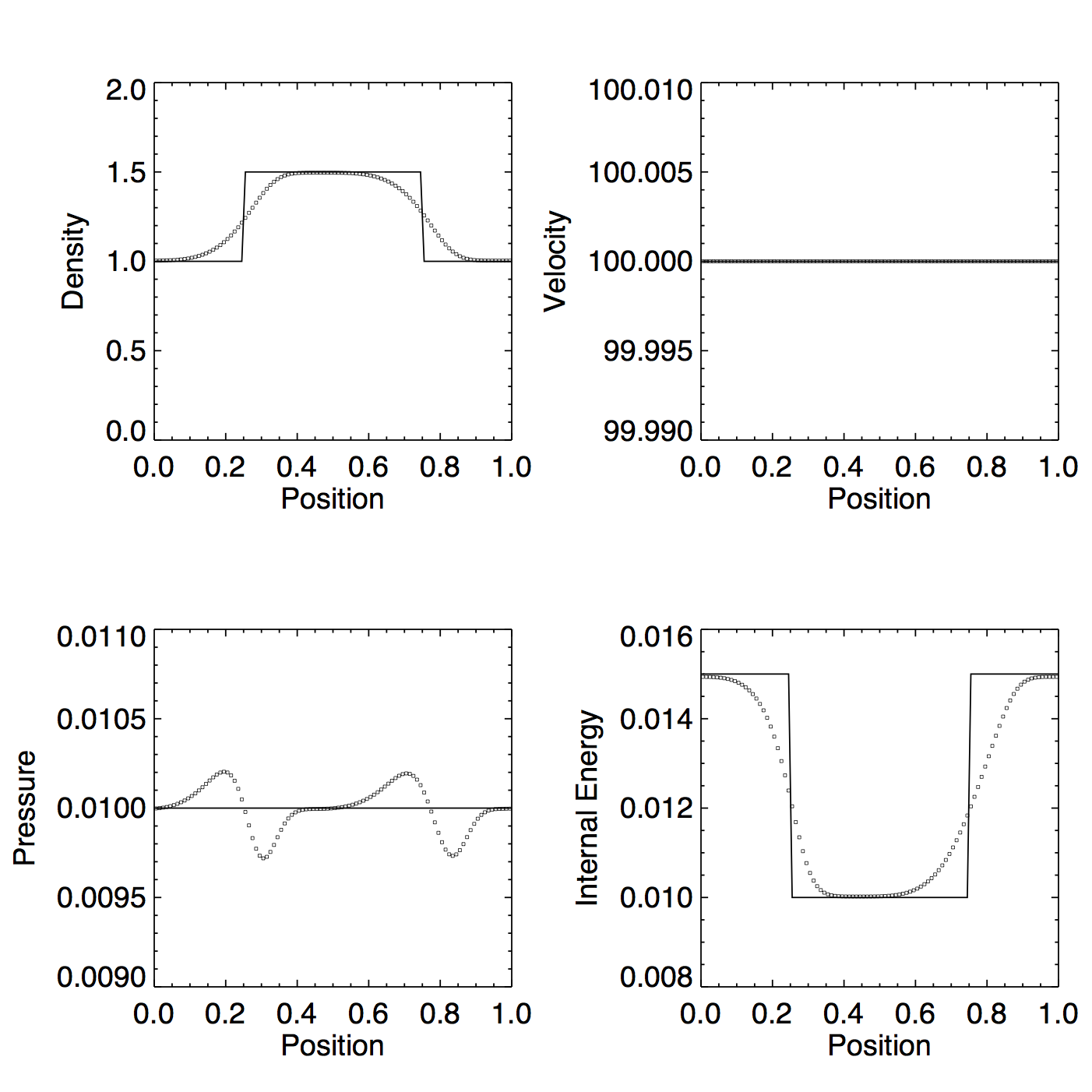# PLM tests (Part 2)

## October 6, 2015

In this post we focus on the high mach number square wave test from previous posts, now trying to answer the question - how do we either a) come up with a better approximation for the internal energy update or b) at least come up with a switch that does not degrade the solution?

Without dual energy, Cholla seems to suffer only from diffusion in these tests, which isn’t a scenario we expect dual energy to improve. However, we certainly don’t want to employ a dual energy method that makes the solution worse:

Figure 1: PLMC, exact solver, no dual energy, $v \approx 800 c_s$Figure 2: Same as Figure 1 but with dual energyNote: Previous versions of Figure 2 looked worse - I found a bug in the internal energy PLMC reconstruction that was allowing non-monotonic reconstructions.

What can we do?

First, what happens if we get rid of the total energy synchronization?

Figure 3: Same as Figure 2 but without the total energy syncNow the internal energy update has no effect on the hydrodynamics, so the perturbations in the velocity disappear. Similalry, the perturbations in the pressure as calculated from the total energy (not shown) also vanish, but the pressure as calculated from the internal energy (shown above) is as bad as Figure 2, or maybe worse. The internal energy is not very different between Figure 2 and Figure 3, despite the fact that the inputs to the internal energy update (the P, $v_{i-1}$, $v_{i+1}$ terms, etc.) should be different now that they are not getting perturbed. This is probably just because the perturbations are small in scale.